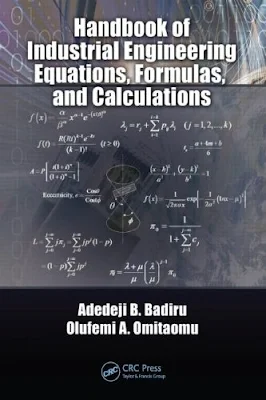# Handbook of Industrial Engineering Equations, Formulas, and Calculations

## Handbook of Industrial Engineering Equations, Formulas, and Calculations

Preference :

Calculations form the basis for engineering practice. Engineering researchers, instructors, students, and practitioners all need simple guides for engineering calculations. Although several books are available in the market for general engineering calculations, none is available directly for industrial engineering calculations. This book is designed to fill that void. It presents a general collection of mathematical equations that are likely to be encountered in the practice of industrial engineering.
Industrial engineering practitioners do not have to be computational experts; they just have to know where to get the computational resources that they need. This book provides access to computational resources needed by industrial engineers. Industrial engineering is one of the most versatile and flexible branches of engineering. It has been said that engineers make things, whereas industrial engineers make things better. To make something better requires an understanding of its basic characteristics. The underlying equations and calculations facilitate that understanding. This book
consists of several sections, each with a focus on a particular problem area. The book
include the following topics:

• Basic math calculations
• Engineering math calculations
• Production engineering calculations
• Engineering economics calculations
• Ergonomics calculations
• Facility layout calculations
• Production sequencing and scheduling calculations
• Systems engineering calculations
• Data engineering calculations
• Project engineering calculations
• Simulation and statistical equations

The book is unique in the market because it is the first book of its kind to focus exclusively on industrial engineering calculations with a correlation to applications for practice.Download Handbook of Industrial Engineering Equations, Formulas, and Calculations

Content :
• Computational Foundations of Industrial Engineering
• Basic Mathematical Calculations
• Statistical Distributions, Methods, and Applications
• Computations with Descriptive Statistics
• Computations for Economic Analysis
• Industrial Production Calculations
• Forecasting Calculations
• Six Sigma and Lean
• Risk Computations
• Computations for Project Analysis
• Product Shape and Geometrical Calculations
• General Engineering Calculations